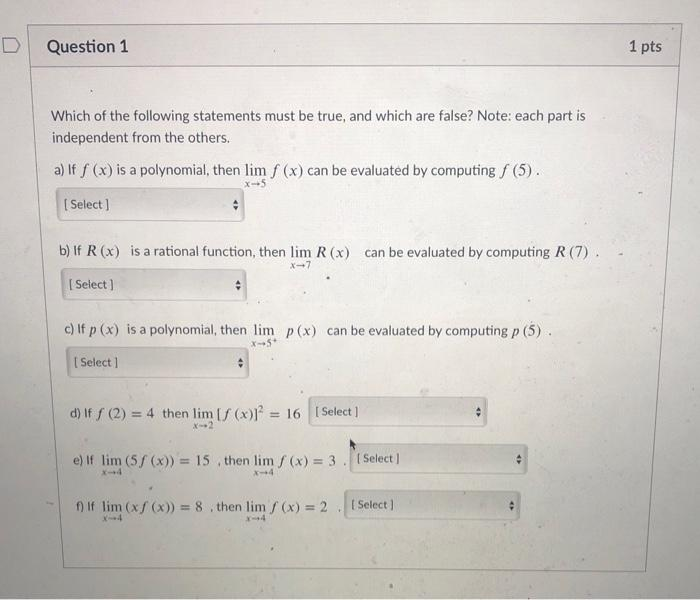# Question Solved1 AnswerD Question 1 1 pts Which of the following statements must be true, and which are false? Note: each part is independent from the others. a) If S (x) is a polynomial, then lim f (x) can be evaluated by computing S (5). Select b) If R(x) is a rational function, then lim R(x) can be evaluated by computing R (7) X-7 Select) c) If p(x) is a polynomial, then lim p(x) can be evaluated by computing p (5) X-5 Select] d) If S (2) = 4 then lim (~) = 16 [Select) e) If lim (58 (x)) = 15 then lim /(x) = 3 . Select fif lim (xf (x)) = 8. then lim / (x) = 2 Select)Transcribed Image Text: D Question 1 1 pts Which of the following statements must be true, and which are false? Note: each part is independent from the others. a) If S (x) is a polynomial, then lim f (x) can be evaluated by computing S (5). Select b) If R(x) is a rational function, then lim R(x) can be evaluated by computing R (7) X-7 Select) c) If p(x) is a polynomial, then lim p(x) can be evaluated by computing p (5) X-5 Select] d) If S (2) = 4 then lim (~) = 16 [Select) e) If lim (58 (x)) = 15 then lim /(x) = 3 . Select fif lim (xf (x)) = 8. then lim / (x) = 2 Select)
More
Transcribed Image Text: D Question 1 1 pts Which of the following statements must be true, and which are false? Note: each part is independent from the others. a) If S (x) is a polynomial, then lim f (x) can be evaluated by computing S (5). Select b) If R(x) is a rational function, then lim R(x) can be evaluated by computing R (7) X-7 Select) c) If p(x) is a polynomial, then lim p(x) can be evaluated by computing p (5) X-5 Select] d) If S (2) = 4 then lim (~) = 16 [Select) e) If lim (58 (x)) = 15 then lim /(x) = 3 . Select fif lim (xf (x)) = 8. then lim / (x) = 2 Select)